## Transistor Sizing

• So far, we have assumed that to get symmetric rise and fall times:• Does this rule reduce overall delay ?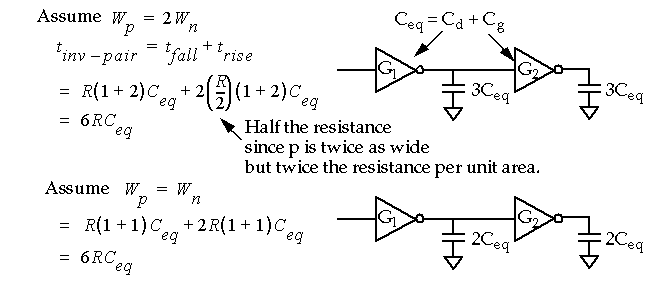• Therefore, in self-loaded circuits (circuits without significant routing capacitance and fanouts), equal sized devices can be used to reduce power dissipation and area without sacrificing performance (overall delay).

## Stage Ratio

• How do we drive large load capacitances, e.g. off-chip wires via the I/O pads, long buses, etc. ?

• By using a chain of inverters, where each successive inverter is larger than the previous one.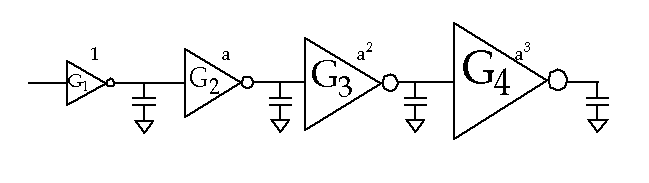• What is the optimal value of a (the stage ratio) that both
• Minimizes the delay through the chain.
• Minimizes the area and power.

• The magic number a is e (~2.7) - see analysis in book.
• The optimal value may vary depending on process parameters.

## Power Dissipation

• Two components of power dissipation in CMOS circuits:
• Static power
• Dynamic power

• Static power dissipation:
• Reverse-bias leakage current through parasitic diodes formed by source/drain diffusion and n-well diffusion.
• Through-current of pseudo-nMOS devices.
• Subthreshold conduction (current that flows when V in < V tn ).
• Becoming more important as power supply is scaled down.

## Static Dissipation

• Reverse-bias leakage current• Total Static Power dissipation:## Dynamic Dissipation

• The current required to charge/discharge capacitive load usually dominates the crowbar (short circuit) current.

• For example: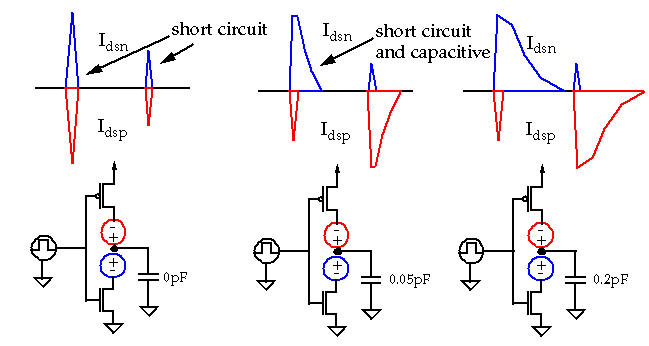## Capacitive Dissipation

• However, slow rise and fall times will increase crowbar current of driven gates.

• Assuming a step input and a repetition frequency of f p , the average dynamic power, P d , is expressed as: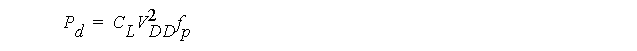• Therefore power is proportional to
• The switching frequency
• But goes up as V DD 2 .

• Also, power is independent of device parameters, such as V t or beta.

## Total Power Dissipation

• P total = P s + P d + P short-circuit (see text P. 236)

• Detailed analysis of power is often impractical.

• Consider the following simplifications:
• Calculate total capacitance driven by the gates in the circuit.
• Estimate the percentage of the devices operating at the max clock frequency (e.g. 50%).
• Use the dynamic power dissipation expression:• Power minimization:
• Use complementary logic gates to reduce through current (static)
• Use minimum-size devices to reduce diffusion leakage (static).
• Reduce V DD , the frequency and the switched capacitance (dynamic).

## Sizing Routing Conductors

• The size of metal conductors is important because:
• Metal migration.
• Power supply noise and integrity.
• RC delay (considered previously).

• Electro-migration is the transport of metal ions through a conductor induced by direct current.

• A 'safe' value of current density, J , is:
•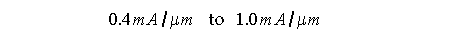•
• For example, consider a clock buffer that drives a 100 pF load at 50 MHz: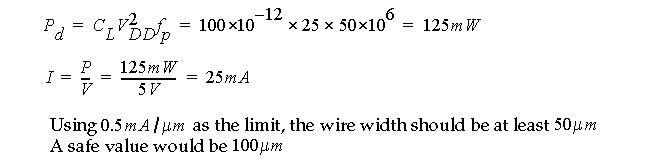## Sizing Routing Conductors

• Power supply noise and integrity:
• IR drops on V DD and V SS (voltage drops due to current spikes and the resistance of the metal) can occur causing gates to fail.

• What is the voltage drop (ground bounce) in the power and ground wires if the buffer is 500 microns from the power and ground pads ?## Design Margining

• Sources of variations that effect nominal circuit behavior (2 environmental, 1 manufacturing):
• Operating Temperature
• Supply Voltage: Data sheets give +/- 10%, e.g., 3.0 to 3.6 for 3.3V.
• Process Variation: Normal to keep parts within 2 or 3 sigma.

• We must design the circuit to operate over all extremes of these variables.

• Temperature :
• What happens to I ds with temperature ?## Design Corners

• Sources of process variation include changes in doping densities, oxide thickness and line width variations.

• The following boundary combinations may result
• Fast-n, Fast-p
• Fast-n, Slow-p
• Slow-n, Fast-p
• Slow-n, Slow-p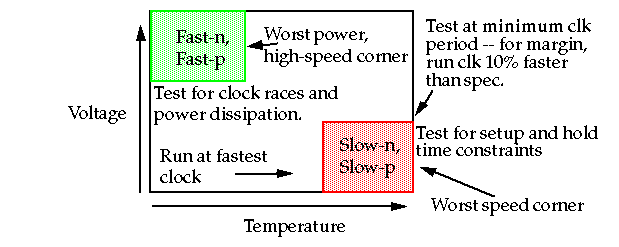## Yield

• Defined as:• Yield verses Testing• Yield is influenced by:
• Technology
• Chip Area
• Layout

## Yield

• A simple model for yield (Seed's model):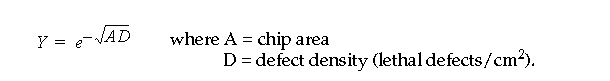• Clearly, yield decreases dramatically as the area of the chip increases.

• Yield and testing are related by• Another exponential function that states that if yield is low, we better have high test coverage (+99%) otherwise we ship lots of bad parts.

• Device testing is a course of its own - stayed tuned.

## First Order Approximations of Scaling

• Constant field scaling : 1/alpha scaling applied to all dimensions, device voltages and concentration densities.
• I ds per transistor scales by 1/alpha.
• # of transistors per unit area scales by alpha 2 .
• Current density scales by alpha, power density remains constant (VI/A),
• e.g., (1/alpha*1/alpha)*alpha 2

• Constant voltage scaling : V DD is held constant while process is scaled.
• I ds per transistor scales by alpha.
• # of transistors per unit area scales up by alpha 2 .
• Current density scales by alpha 3 , power density scales by alpha 3 .

• Lateral scaling : Only the gate length is scaled ( gate-shrink ).
• I ds per transistor scales by alpha.
• # of transistors per unit area scales by alpha.
• Current density scales by alpha 2 , power density scales by alpha 2 .